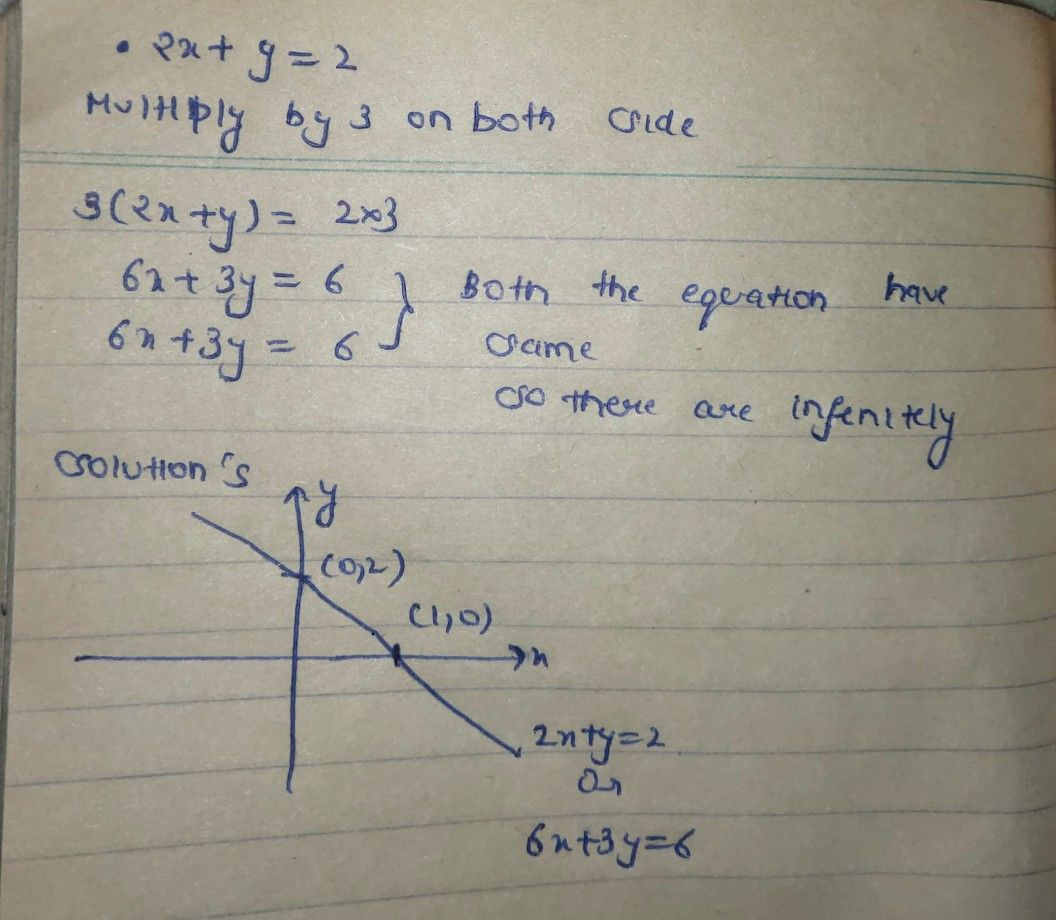Symbol
Problem$\begin{cases} 2x+y=2 \\ 6x+3y=6 \end{cases}$
Other
SolutionStudent
the writing of the words is a bit confusing can u type the explanation tnxmultiply by 3 on both side
both the equation have same so they have infinitely solutionStudent
waiiit the explanation is a bit off can u change the grammar a bit? appreciate it ☺️
the second explenations grammar is a bit ofd
off